# Work with rectangles¶

The rectangle is a very useful object in graphics programming. It has its own Rect class in Pygame and is used to store and manipulate a rectangular area. A Rect object can be created by giving:

• the 4 parameters left, top, width and height

• the position and size

• an object which has a rect attribute

Rect(left, top, width, height)
Rect(pos, size)
Rect(obj)


A function which expects a Rect argument accepts equally one of the three above values. Methods which change the position or size, such as move() and inflate() leave the original Rect untouched and return a new Rect. They also have the in place version move_ip and inflate_ip which act upon the original Rect.

## Virtual attributes¶

The Rect object has several virtual attributes which can be used to move and align the Rect. Assignment to these attributes just moves the rectangle without changing its size:

x, y
top, left, bottom, right
topleft, bottomleft, topright, bottomright
midtop, midleft, midbottom, midright
center, centerx, centery


The assignment of these 5 attributes changes the size of the rectangle, by keeping its top left position.

size, width, height, w, h


The following program prints these virtual attributes to the console:

x=50, y=60, w=200, h=80
left=50, top=60, right=250, bottom=140
center=(150, 100)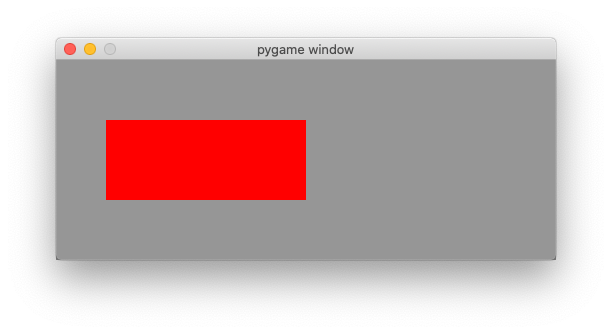import pygame
from pygame.locals import *

SIZE = 500, 200
RED = (255, 0, 0)
GRAY = (150, 150, 150)

pygame.init()
screen = pygame.display.set_mode(SIZE)

rect = Rect(50, 60, 200, 80)
print(f'x={rect.x}, y={rect.y}, w={rect.w}, h={rect.h}')
print(f'left={rect.left}, top={rect.top}, right={rect.right}, bottom={rect.bottom}')
print(f'center={rect.center}')

running = True
while running:
for event in pygame.event.get():
if event.type == QUIT:
running = False

screen.fill(GRAY)
pygame.draw.rect(screen, RED, rect)
pygame.display.flip()

pygame.quit()


rect1.py

## Points of interest¶

The Rect class defines 4 cornerpoints, 4 mid points and 1 centerpoint.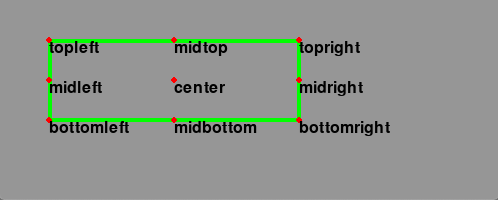from rect import *

pts = ('topleft', 'topright', 'bottomleft', 'bottomright',
'midtop', 'midright', 'midbottom', 'midleft', 'center')

running = True
while running:
for event in pygame.event.get():
if event.type == QUIT:
running = False

screen.fill(GRAY)
pygame.draw.rect(screen, GREEN, rect, 4)
for pt in pts:
pos = eval('rect.'+pt)
draw_text(pt, pos)
pygame.draw.circle(screen, RED, pos, 3)

pygame.display.flip()

pygame.quit()


rect2.py

## Horizontal and vertical alignment¶

In the following example we use 3 keys to align a rectangle horizontally:

• L - left
• C - center
• R - right

and 3 other keys to align the rectangle vertically:

• T - top
• M - middle
• B - bottom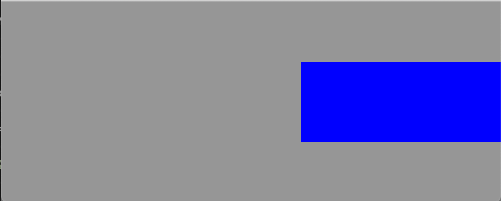from rect import *

rect = Rect(50, 60, 200, 80)

while running:
for event in pygame.event.get():
if event.type == QUIT:
running = False

if event.type == KEYDOWN:
if event.key == K_l:
rect.left = 0
if event.key == K_c:
rect.centerx = width//2
if event.key == K_r:
rect.right = width

if event.key == K_t:
rect.top = 0
if event.key == K_m:
rect.centery = height//2
if event.key == K_b:
rect.bottom = height

screen.fill(GRAY)
pygame.draw.rect(screen, BLUE, rect)
pygame.display.flip()

pygame.quit()


rect3.py

## Move a rectangle with keys¶

The method move(v) creates a new Rect which has moved by a vector v. The method move_ip(v) moves a Rect in place. The following program uses the 4 arrow keys to move a rectangle around. The thin blue rectangle is the orignal one, the thick red rectangle is the moved one.

We use a dictionary to associate a motion vector to each of the 4 arrow keys. For each direction the movement is by 5 pixels:

dir = {K_LEFT: (-5, 0), K_RIGHT: (5, 0), K_UP: (0, -5), K_DOWN: (0, 5)}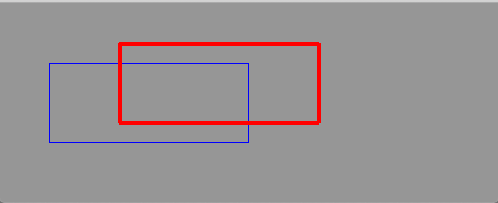from rect import *

rect0 = Rect(50, 60, 200, 80)
rect = rect0.copy()

while running:
for event in pygame.event.get():
if event.type == QUIT:
running = False

if event.type == KEYDOWN:
if event.key in dir:
v = dir[event.key]
rect.move_ip(v)

screen.fill(GRAY)
pygame.draw.rect(screen, BLUE, rect0, 1)
pygame.draw.rect(screen, RED, rect, 4)
pygame.display.flip()

pygame.quit()


rect4.py

## Inflate a rectangle¶

The method inflate(v) grows or shrinks a rectangle by a vector v and creates a new Rect. The method inflate_ip(v) grows or shrinks a Rect in place. The following program uses the 4 arrow keys to change the size of a rectangle. The thin blue rectangle is the orignal one, the thick red rectangle is the changed one.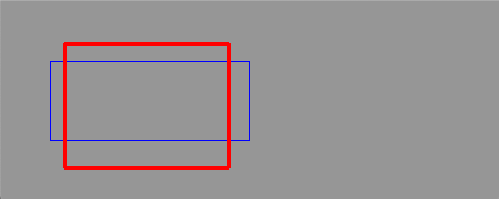from rect import *

rect0 = rect.copy()

while running:
for event in pygame.event.get():
if event.type == QUIT:
running = False

if event.type == KEYDOWN:
if event.key in dir:
v = dir[event.key]
rect.inflate_ip(v)

screen.fill(GRAY)
pygame.draw.rect(screen, BLUE, rect0, 1)
pygame.draw.rect(screen, RED, rect, 4)
pygame.display.flip()

pygame.quit()


rect5.py

## Clip a rectangle¶

The method r0.clip(r1) returns a new rectangle which is the intersection of the two rectangles. The method r0.union(r1) returns a new rectangle which is the union of the two rectangles.

The program belows shows two rectangles in red and blue outline. The green rectangle is the clipped area (intersection). The yellow rectangle is the union of the two rectangles.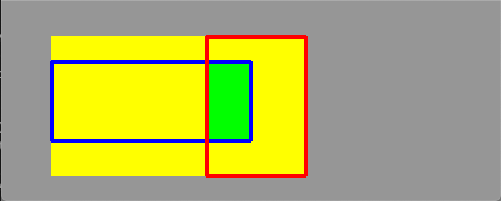from rect import *

r0 = Rect(50, 60, 200, 80)
r1 = Rect(100, 20, 100, 140)

while running:
for event in pygame.event.get():
if event.type == QUIT:
running = False

if event.type == KEYDOWN:
if event.key in dir:
r1.move_ip(dir[event.key])

clip = r0.clip(r1)
union = r0.union(r1)

screen.fill(GRAY)
pygame.draw.rect(screen, YELLOW, union, 0)
pygame.draw.rect(screen, GREEN, clip, 0)
pygame.draw.rect(screen, BLUE, r0, 4)
pygame.draw.rect(screen, RED, r1, 4)
pygame.display.flip()

pygame.quit()


rect6.py

## Move a rectangle with the mouse¶

The function rect.collidepoint(pos) returns True if the point collides with the rectangle. We use it with the mouse position to check if the mouse click has happened inside the rectangle. If that is the case, we move the rectangle by the relative motion of the mouse event.rel.

The boolean variable moving is set when the mouse button goes down inside the rectangle. It remains True until the button goes up again. The rectangle is only moved when the mouse click has happened inside the rectangle. While the rectangle is moving, we add a blue outline.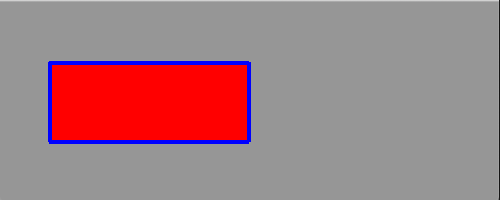from rect import *

moving = False

while running:
for event in pygame.event.get():
if event.type == QUIT:
running = False

elif event.type == MOUSEBUTTONDOWN:
if rect.collidepoint(event.pos):
moving = True

elif event.type == MOUSEBUTTONUP:
moving = False

elif event.type == MOUSEMOTION and moving:
rect.move_ip(event.rel)

screen.fill(GRAY)
pygame.draw.rect(screen, RED, rect)
if moving:
pygame.draw.rect(screen, BLUE, rect, 4)
pygame.display.flip()

pygame.quit()


rect7.py

## A self-moving a rectangle¶

The following code moves a rectangle by the amount v:

rect.move_ip(v)


It then checks the 4 borders and inverts the speed component if the rectangle is outside of the application window.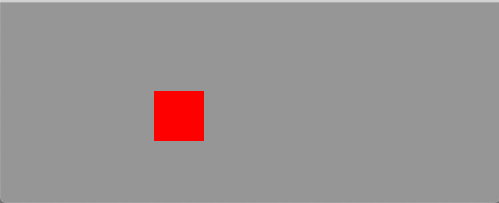from rect import *

rect = Rect(100, 50, 50, 50)
v = [2, 2]

while running:
for event in pygame.event.get():
if event.type == QUIT:
running = False

rect.move_ip(v)

if rect.left < 0:
v *= -1
if rect.right > width:
v *= -1
if rect.top < 0:
v *= -1
if rect.bottom > height:
v *= -1

screen.fill(GRAY)
pygame.draw.rect(screen, RED, rect)
pygame.display.flip()

pygame.quit()


rect8.py

## Colliding points¶

The method rect.collidepoint(p) checks if a rectangle rect collides with point p. In the following program we create 100 random points and color them red if they fall inside the rectangle.

Each time the R key is pressed 100 new random points are created.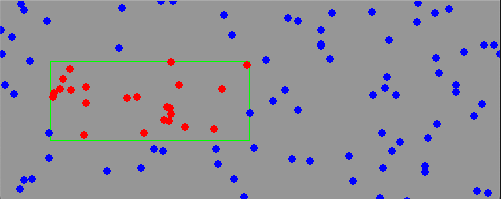from rect import *

points = random_points(100)

while running:
for event in pygame.event.get():
if event.type == QUIT:
running = False

if event.type == KEYDOWN:
if event.key == K_r:
points = random_points(100)

screen.fill(GRAY)
pygame.draw.rect(screen, GREEN, rect, 1)
for p in points:
if rect.collidepoint(p):
pygame.draw.circle(screen, RED, p, 4, 0)
else:
pygame.draw.circle(screen, BLUE, p, 4, 0)

pygame.display.flip()

pygame.quit()


rect9.py

## Colliding rectangles¶

The method rect.colliderect(r) checks if a rectangle rect collides with another rectangle r. In the following program we create 50 random rectangles and color them red if they collide with the green rectangle.

Each time the R key is pressed 50 new random rectangles are created.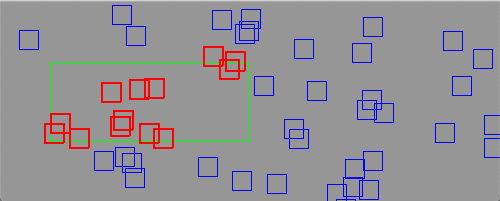from rect import *

n = 50
rects = random_rects(n)

while running:
for event in pygame.event.get():
if event.type == QUIT:
running = False

if event.type == KEYDOWN:
if event.key == K_r:
rects = random_rects(n)

screen.fill(GRAY)
pygame.draw.rect(screen, GREEN, rect, 1)

for r in rects:
if rect.colliderect(r):
pygame.draw.rect(screen, RED, r, 2)
else:
pygame.draw.rect(screen, BLUE, r, 1)

pygame.display.flip()

pygame.quit()


rect10.py

## Overlapping rectangles¶

The method rect.colliderect(r) checks if a rectangle rect collides with another rectangle r. If we want to know if there are any two overlapping rectangles, then we have to compare each rectangle with each other one. The number of comparisons increases as power of 2.

Each time the R key is pressed 20 new random rectangles are created.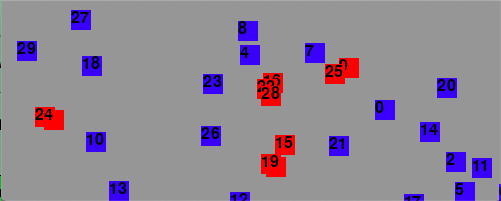from rect import *

n = 30
rects = random_rects(n)

while running:
for event in pygame.event.get():
if event.type == QUIT:
running = False

if event.type == KEYDOWN:
if event.key == K_r:
rects = random_rects(n)

screen.fill(GRAY)

intersecting = []
for i in range(n-1):
r0 = rects[i]
for j in range(i+1, n):
r1 = rects[j]
if r0.colliderect(r1):
intersecting.append(r0)
intersecting.append(r1)
break

for i, r in enumerate(rects):
color = RED if r in intersecting else BLUE
pygame.draw.rect(screen, color, r)
draw_text(str(i), r.topleft)

pygame.display.flip()

pygame.quit()


rect11.py

## The common code¶

The common has been placed to a separate file:

import pygame
from pygame.locals import *
from random import randint

width = 500
height = 200

RED = (255, 0, 0)
GREEN = (0, 255, 0)
BLUE = (0, 0, 255)

YELLOW = (255, 255, 0)
MAGENTA = (255, 0, 255)
CYAN = (0, 255, 255)

BLACK = (0, 0, 0)
GRAY = (150, 150, 150)
WHITE = (255, 255, 255)

dir = {K_LEFT: (-5, 0), K_RIGHT: (5, 0), K_UP: (0, -5), K_DOWN: (0, 5)}
rect = Rect(50, 60, 200, 80)

def draw_text(text, pos):
img = font.render(text, True, BLACK)
screen.blit(img, pos)

def random_point():
x = randint(0, width)
y = randint(0, height)
return (x, y)

def random_points(n):
points = []
for i in range(n):
p = random_point()
points.append(p)
return points

def random_rects(n):
rects = []
for i in range(n):
r = Rect(random_point(), (20, 20))
rects.append(r)
return rects

pygame.init()
screen = pygame.display.set_mode((width, height))
clock = pygame.time.Clock()
font = pygame.font.Font(None, 24)
running = True


rect.py